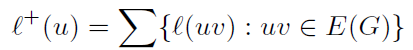##### Current Development of Historical Methods for Solving Linear Systems of Simultaneous Congruences

Yang Liu, Robert Goutte

Journal of Advances in Mathematics and Computer Science, Page 265-273
DOI: 10.9734/BJMCS/2015/16683

Based on the very old methods of solving linear systems of simultaneous congruences, the Chinese Remainder Theorem is still an irreplaceable tool in Computing, Signal Processing and Information security.
We demonstrate its potential by considering an example of an application concerning quaternionic imaging.

##### I-pure Submodules, I-FP-injective Modules and I-flat Modules

Zhanmin Zhu

Journal of Advances in Mathematics and Computer Science, Page 170-188
DOI: 10.9734/BJMCS/2015/16451

Let R be a ring and I an ideal of R. We define and study I-pure submodules , I-FP-injective modules, I-flat modules , I-coherent rings and I-semihereditary rings. Using the concepts of I-FP-injectivity and I-flatness of modules, we also present some characterizations of I-coherent rings and I-semihereditary rings.

##### V4- Magic Labelings of Some Wheel Related Graphs

P. T. Vandana, V. Anil Kumar

Journal of Advances in Mathematics and Computer Science, Page 189-219
DOI: 10.9734/BJMCS/2015/16439

The Klein 4-group, denoted by V4 is an abelian group of order 4. It has elements V= {0, a, b, c}, with a + a = b + b = c + c = 0 and a + b = c, b + c = a, c + a = b. A graph G= (V (G), E(G)), with vertex set V (G) and edge set E(G), is said to be V4 magic if there exists a labeling l : E(G) → V4 \ {0} such that the induced vertex labeling l+: V (G) → V4 deﬁned byis a constant map. If this constant is equal to a, we say that l is an a-sum V4 magic labeling of G. Any graph that admits an a- sum V4 magic labeling is called an a- magic V graph. When this constant is 0 we call G a zero-sum V4 -magic graph. We divide the class of V4 magic graphs into the following three categories:

(i) Va, the class of a-sum V4 magic graphs,

(ii) V0, the class of zero-sum V4 magic graphs,

(iii) Va;04, the class of graphs which are both a-sum and zero -sum V4 magic.

In this paper, we identify some cycle related graphs which belong to the above categories.

##### On Non-metric Covering Lemmas and Extended Lebesgue-differentiation Theorem

Mangatiana A. Robdera

Journal of Advances in Mathematics and Computer Science, Page 220-228
DOI: 10.9734/BJMCS/2015/16752

We give a non-metric version of the Besicovitch Covering Lemma and an extension of the Lebesgue-Differentiation Theorem into the setting of the integration theory of vector valued functions.

##### Quartic Cyclic Homogeneous Polynomial Inequalities of Three Nonnegative Variables

Yuanzhe Zhou

Journal of Advances in Mathematics and Computer Science, Page 229-237
DOI: 10.9734/BJMCS/2015/16723

We present and prove a set of necessary and sufficient conditions that the inequality f4(x, y, z) ≥ 0 holds for all nonnegative real variables x, y, z, where f4(x, y, z) is a cyclic homogeneous polynomial of degree four which satisfies f4(1, 1, 1) = 0.

##### Solving Investment Problem with Inexact Rough Interval Data through Dynamic Programming Approach

E. E. Ammar, H. A. Khalifa

Journal of Advances in Mathematics and Computer Science, Page 238-245
DOI: 10.9734/BJMCS/2015/15849

Finding the best investment is an interesting optimization problem. When we deal with such a problem in an uncertain and vague environment, the optimization problem becomes more difficult. In this paper, we deal with rough data expressed in the form of an inexact rough interval fuzzy numbers, and then solve the optimization problem for atypical investment problem to obtain a rough interval solution. The process of optimization is illustrated by a numerical example.

##### A Mathematical Model for Population Density Dynamics of Weed-Crop Competition

M. O. Nasir, N. I. Akinwande, M. G. M. Kolo, J. Mohammed, Rose Abbah

Journal of Advances in Mathematics and Computer Science, Page 246-264
DOI: 10.9734/BJMCS/2015/15975

A model for the dynamics of homogeneous population competition between two species of weeds and a crop is formulated to gain in-sight into the behaviour of crop growing with weeds. We used assumptions based upon reasonable biological process to derive from single weed model equation, the systems of difference equations that described the dynamics of weed-crop competition. Steady- state solutions of the model are obtained and analyzed for local stability or otherwise. The results show that the extinction steady state is not stable without control and the conditions for stability of two plants steady-states are given. The weed-crop coexistence steady state of our model is locally asymptotically stable. Besides, the graphical profile of the model shows that the crop’s growth may be stagnated by the weeds’ densities. Hence, we conclude that the crop’s growth may be stagnated, but survive in the mixture of the two species of weeds. However, application of effective control measure to eradicate the two weeds species will enhance crop growth at its optimum capacity.